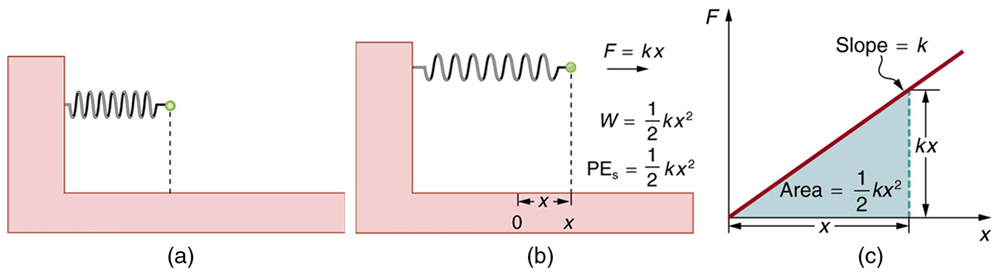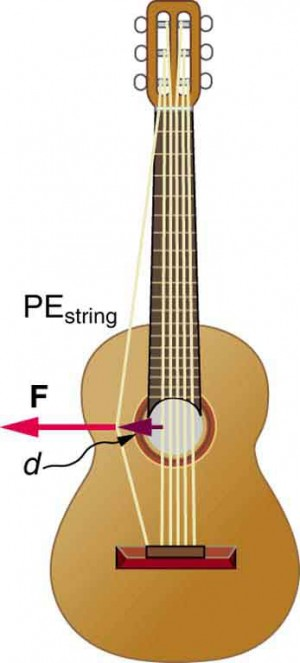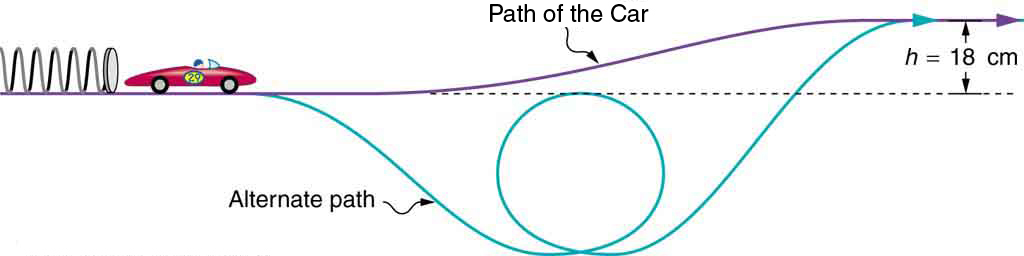## Conservative Forces and Potential Energy

### Learning Objectives

By the end of this section, you will be able to:

• Define conservative force, potential energy, and mechanical energy.
• Explain the potential energy of a spring in terms of its compression when Hooke’s law applies.
• Use the work-energy theorem to show how having only conservative forces implies conservation of mechanical energy.

## Potential Energy and Conservative Forces

Work is done by a force, and some forces, such as weight, have special characteristics. A conservative force is one, like the gravitational force, for which work done by or against it depends only on the starting and ending points of a motion and not on the path taken. We can define a potential energy (PE) for any conservative force, just as we did for the gravitational force. For example, when you wind up a toy, an egg timer, or an old-fashioned watch, you do work against its spring and store energy in it. (We treat these springs as ideal, in that we assume there is no friction and no production of thermal energy.) This stored energy is recoverable as work, and it is useful to think of it as potential energy contained in the spring. Indeed, the reason that the spring has this characteristic is that its force is conservative. That is, a conservative force results in stored or potential energy. Gravitational potential energy is one example, as is the energy stored in a spring. We will also see how conservative forces are related to the conservation of energy.

### Potential Energy and Conservative Forces

Potential energy is the energy a system has due to position, shape, or configuration. It is stored energy that is completely recoverable.

A conservative force is one for which work done by or against it depends only on the starting and ending points of a motion and not on the path taken.

We can define a potential energy (PE) for any conservative force. The work done against a conservative force to reach a final configuration depends on the configuration, not the path followed, and is the potential energy added.

## Potential Energy of a Spring

First, let us obtain an expression for the potential energy stored in a spring (PEs). We calculate the work done to stretch or compress a spring that obeys Hooke’s law. (Hooke’s law was examined in Elasticity: Stress and Strain, and states that the magnitude of force F on the spring and the resulting deformation ΔL are proportional, kΔL.) (See Figure 1.) For our spring, we will replace ΔL (the amount of deformation produced by a force F) by the distance x that the spring is stretched or compressed along its length. So the force needed to stretch the spring has magnitude Fkx, where k is the spring’s force constant. The force increases linearly from 0 at the start to kx in the fully stretched position. The average force is $\frac{kx}{2}\\$. Thus the work done in stretching or compressing the spring is $W_\text{s}=Fd=\left(\frac{kx}{2}\right)x=\frac{1}{2}kx^2\\$. Alternatively, we noted in Kinetic Energy and the Work-Energy Theorem that the area under a graph of F vs. x is the work done by the force. In Figure 1c we see that this area is also $\frac{1}{2}kx^2\\$. We therefore define the potential energy of a spring, PEs, to be

$\text{PE}_{\text{s}}=\frac{1}{2}kx^2\\$,

where k is the spring’s force constant and x is the displacement from its undeformed position. The potential energy represents the work done on the spring and the energy stored in it as a result of stretching or compressing it a distance x. The potential energy of the spring PEs does not depend on the path taken; it depends only on the stretch or squeeze x in the final configuration.Figure 1. (a) An undeformed spring has no PEs stored in it. (b) The force needed to stretch (or compress) the spring a distance x has a magnitude F = kx, and the work done to stretch (or compress) it is $\frac{1}{2}kx^2\\$. Because the force is conservative, this work is stored as potential energy (PEs) in the spring, and it can be fully recovered. (c) A graph of F vs. x has a slope of k, and the area under the graph is $\frac{1}{2}kx^2\\$. Thus the work done or potential energy stored is $\frac{1}{2}kx^2\\$.

The equation $\text{PE}_{\text{s}}=\frac{1}{2}kx^2\\$ has general validity beyond the special case for which it was derived. Potential energy can be stored in any elastic medium by deforming it. Indeed, the general definition of potential energy is energy due to position, shape, or configuration. For shape or position deformations, stored energy is $\text{PE}_{\text{s}}=\frac{1}{2}kx^2\\$, where k is the force constant of the particular system and x is its deformation. Another example is seen in Figure 2 for a guitar string.Figure 2. Work is done to deform the guitar string, giving it potential energy. When released, the potential energy is converted to kinetic energy and back to potential as the string oscillates back and forth. A very small fraction is dissipated as sound energy, slowly removing energy from the string.

## Conservation of Mechanical Energy

Let us now consider what form the work-energy theorem takes when only conservative forces are involved. This will lead us to the conservation of energy principle. The work-energy theorem states that the net work done by all forces acting on a system equals its change in kinetic energy. In equation form, this is $W_{\text{net}}=\frac{1}{2}mv^2-\frac{1}{2}mv_0^2=\Delta\text{KE}\\$.

If only conservative forces act, then WnetWcwhere Wc is the total work done by all conservative forces. Thus, Wc = ΔKE.

Now, if the conservative force, such as the gravitational force or a spring force, does work, the system loses potential energy. That is, Wc=−ΔPE. Therefore, −ΔPE = ΔKE or ΔKE + ΔPE = 0.

This equation means that the total kinetic and potential energy is constant for any process involving only conservative forces. That is,

$\text{(conservative forces only),}\begin{cases}\text{KE}+\text{PE}=\text{constant}\\\text{or}\\\text{KE}_{\text{i}}+\text{PE}_{\text{i}}=\text{KE}_{\text{f}}+\text{PE}_{\text{f}}\end{cases}\\$

where i and f denote initial and final values. This equation is a form of the work-energy theorem for conservative forces; it is known as the conservation of mechanical energy principle. Remember that this applies to the extent that all the forces are conservative, so that friction is negligible. The total kinetic plus potential energy of a system is defined to be its mechanical energy, (KE+PE). In a system that experiences only conservative forces, there is a potential energy associated with each force, and the energy only changes form between KE and the various types of PE, with the total energy remaining constant.

### Example 1. Using Conservation of Mechanical Energy to Calculate the Speed of a Toy Car

A 0.100-kg toy car is propelled by a compressed spring, as shown in Figure 3. The car follows a track that rises 0.180 m above the starting point. The spring is compressed 4.00 cm and has a force constant of 250.0 N/m. Assuming work done by friction to be negligible, find the following:

1. How fast is the car going before it starts up the slope?
2. How fast is it going at the top of the slope?Figure 3. A toy car is pushed by a compressed spring and coasts up a slope. Assuming negligible friction, the potential energy in the spring is first completely converted to kinetic energy, and then to a combination of kinetic and gravitational potential energy as the car rises. The details of the path are unimportant because all forces are conservative—the car would have the same final speed if it took the alternate path shown.

#### Strategy

The spring force and the gravitational force are conservative forces, so conservation of mechanical energy can be used. Thus,

KEi + PEi = KEf + PEf

or

$\frac{1}{2}{mv_{\text{i}}}^2+mgh_{\text{i}}+\frac{1}{2}{kx_{\text{i}}}^2=\frac{1}{2}{mv_{\text{f}}}^2+mgh_{\text{f}}+\frac{1}{2}{kx_{\text{f}}}^2\\$,

where h is the height (vertical position) and x is the compression of the spring. This general statement looks complex but becomes much simpler when we start considering specific situations. First, we must identify the initial and final conditions in a problem; then, we enter them into the last equation to solve for an unknown.

#### Solution for Part 1

This part of the problem is limited to conditions just before the car is released and just after it leaves the spring. Take the initial height to be zero, so that both hi and hf are zero. Furthermore, the initial speed vi is zero and the final compression of the spring xf is zero, and so several terms in the conservation of mechanical energy equation are zero and it simplifies to

$\frac{1}{2}{kx_{\text{i}}}^2=\frac{1}{2}{mv_{\text{f}}}^2\\$.

In other words, the initial potential energy in the spring is converted completely to kinetic energy in the absence of friction. Solving for the final speed and entering known values yields

$\begin{array}{lll}v_{\text{f}}&=&\sqrt{\frac{k}{m}x_{\text{i}}}\\\text{ }&=&\sqrt{\frac{250.0\text{ N/m}}{0.100\text{ kg}}}\left(0.0400\text{ m}\right)\\\text{ }&=&2.00\text{ m/s}\end{array}\\$

#### Solution for Part 2

One method of finding the speed at the top of the slope is to consider conditions just before the car is released and just after it reaches the top of the slope, completely ignoring everything in between. Doing the same type of analysis to find which terms are zero, the conservation of mechanical energy becomes

$\frac{1}{2}{kx_{\text{i}}}^2=\frac{1}{2}{mv_{\text{f}}}^2+mgh_{\text{f}}\\$.

This form of the equation means that the spring’s initial potential energy is converted partly to gravitational potential energy and partly to kinetic energy. The final speed at the top of the slope will be less than at the bottom. Solving for vf and substituting known values gives

$\begin{array}{lll}v_{\text{f}}&=&\sqrt{\frac{kx_{\text{i}}^2}{m}-2gh_{\text{f}}}\\\text{ }&=&\sqrt{\left(\frac{250.0\text{ N/m}}{0.100\text{ kg}}\right)\left(0.0400\text{ m}\right)^2-2\left(9.80\text{ m/s}^2\right)\left(0.180\text{ m}\right)}\\\text{ }&=&0.687\text{ m/s}\end{array}\\$

#### Discussion

Another way to solve this problem is to realize that the car’s kinetic energy before it goes up the slope is converted partly to potential energy—that is, to take the final conditions in part 1 to be the initial conditions in part 2.

Note that, for conservative forces, we do not directly calculate the work they do; rather, we consider their effects through their corresponding potential energies, just as we did in Example 1. Note also that we do not consider details of the path taken—only the starting and ending points are important (as long as the path is not impossible). This assumption is usually a tremendous simplification, because the path may be complicated and forces may vary along the way.

## PhET Explorations: Energy Skate Park

Learn about conservation of energy with a skater dude! Build tracks, ramps and jumps for the skater and view the kinetic energy, potential energy and friction as he moves. You can also take the skater to different planets or even space!

## Section Summary

• A conservative force is one for which work depends only on the starting and ending points of a motion, not on the path taken.
• We can define potential energy (PE) for any conservative force, just as we defined PEg for the gravitational force.
• The potential energy of a spring is ${\text{PE}}_{s}=\frac{1}{2}{\text{kx}}^{2}\\$, where k is the spring’s force constant and x is the displacement from its undeformed position.
• Mechanical energy is defined to be KE + PE for a conservative force.
• When only conservative forces act on and within a system, the total mechanical energy is constant. In equation form,
$\begin{cases}\text{KE}+\text{PE}=\text{constant}\\\text{or}\\\text{KE}_{\text{i}}+\text{PE}_{\text{i}}=\text{KE}_{\text{f}}+\text{PE}_{\text{f}}\end{cases}\\$
where i and f denote initial and final values. This is known as the conservation of mechanical energy.

### Conceptual Questions

1. What is a conservative force?
2. The force exerted by a diving board is conservative, provided the internal friction is negligible. Assuming friction is negligible, describe changes in the potential energy of a diving board as a swimmer dives from it, starting just before the swimmer steps on the board until just after his feet leave it.
3. Define mechanical energy. What is the relationship of mechanical energy to nonconservative forces? What happens to mechanical energy if only conservative forces act?
4. What is the relationship of potential energy to conservative force?

### Problems & Exercises

1. A 5.00 × 105-kg subway train is brought to a stop from a speed of 0.500 m/s in 0.400 m by a large spring bumper at the end of its track. What is the force constant k of the spring?
2. A pogo stick has a spring with a force constant of 2.50 × 104 N/m, which can be compressed 12.0 cm. To what maximum height can a child jump on the stick using only the energy in the spring, if the child and stick have a total mass of 40.0 kg? Explicitly show how you follow the steps in the Problem-Solving Strategies for Energy.

## Glossary

conservative force: a force that does the same work for any given initial and final configuration, regardless of the path followed

potential energy: energy due to position, shape, or configuration

potential energy of a spring: the stored energy of a spring as a function of its displacement; when Hooke’s law applies, it is given by the expression $\frac{1}{2}{\text{kx}}^{2}\\$ where x is the distance the spring is compressed or extended and k is the spring constant

conservation of mechanical energy: the rule that the sum of the kinetic energies and potential energies remains constant if only conservative forces act on and within a system

mechanical energy: the sum of kinetic energy and potential energy

### Selected Solutions to Problems & Exercises

1. 7.81 × 105 N/m